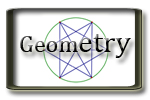G.P. Geometry Unit 6 Relationships Within Triangles Jump to Unit 1 - 2 - 3 - 4 - 5 - 6 - 7- 8- 9 - 10 - 11 Unit 6 Textbook with Examples and extra problems. Big Ideas Video Tutorials - Lots of video examples

#Lesson

Handouts/Activities

Videos and Support Resources

6.1 Perpendiculandar and Angle Bisectors
 Example 1 Example 2 Example 3 Example 4 Example 5
6.2 Bisectors of Triangles

GSP

 Example 1 Example 2 Example 3 Example 4
6.3 Medians and Altitudes of Triangles
 Example 1 Example 2 Example 3 Example 4
6.4 The Triangle Midsegment Theorem
 Example 1 Example 2 Example 3 Example 4 Example 5
6.5 Indirect Proof and Inequalities in One Triangle
 Example 1 Example 2 Example 3 Example 4 Example 5
6.6 Inequalities in Two Triangles
 Example 1 Example 2 Example 3 Example 4 Example 5
Test Day Test Day Instructions